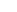# Aapo Hyvärinen## ISCTEST: Testing Independent Components

This page contains Matlab code for testing which independent components are statistically significant. The code assumes you have computed ICA for several datasets. In general, you can just split your dataset into two and do ICA separately on those two set. In a neuroimaging context, you perform ICA separately on several subjects or several segments (sessions) of data from a single subject.

The code then compares and clusters the independent components for the different datasets (perhaps subjects or sessions) and determines which are found more consistently (i.e. which are more similar across subjects or sessions) than expected by chance.

First, you have to choose if you want to test the significance of the consistency based on the mixing matrix or the independent components themselves.

Testing mixing matrix: A simple example of using the code:

`clustering=isctest(Atensor,0.05,0.05,'mixing');`

where Atensor is a three-dimensional tensor such that Atensor(:,:,k) contains the mixing matrix estimated from the k-th subject or session. With this option, algorithm considers two components to be similar if the corresponding columns of the mixing matrix are similar. Use this for temporal ICA, as typical in EEG/MEG.

Testing independent component patterns: A simple example of using the code:

`clustering=isctest(Stensor,0.05,0.05,'components');`

where Stensor is a three-dimensional tensor such that Stensor(:,:,k) contains the independent components estimated from the k-th subject or session (the transpose of the matrix S given by FastICA). With this option, we consider two components to be similar if the values of the independent components themselves are similar. Use this, e.g., for spatial ICA of fMRI to test similarity of spatial patterns.

Note that in both cases you have to do ICA first, this code does not include ICA estimation. (You must used ordinary ICA here, e.g. using the FastICA package. Do not use the ICASSO package which is not compatible with ISCTEST.)

The variable clustering will have each cluster of consistent components as one of its rows, the columns corresponding to the subjects, and the entries giving the indices of the components belonging to the cluster (zero meaning no component belongs to this cluster). For example, suppose you have four subjects/sessions and clustering has the following row:

`[ 5, 3, 4, 0 ]`
This means that the method found a cluster of consistent components which contains the 5th component of subject #1, the 3rd component of subject #2, the 4th components of subject #3, but no component from subject #4 fitted this particular cluster. Each row should be interpreted separately as one cluster of consistent components.

For the theory, see the papers
Testing the ICA mixing matrix based on inter-subject or inter-session consistency (for testing the mixing matrix)
Testing independent component patterns by inter-subject or inter-session consistency (for testing the independent components).

(For version control and historical reference, here is the first version of the method, including testing mixing matrix only as in the first paper above.)